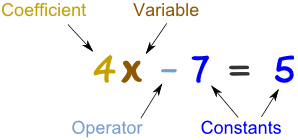# Math 10 Week 8

This week we started polynomials. The first time you look at polynomials you will probably have a heart attack. But they’re not as hard as you think. In this post I will teach you what a polynomial is and some vocabulary as well as how to do a question.

Vocabulary:

Type– Types of polynomials

Monomial- Consisting of one term.

Binomial- An algebraic expression of the sum or the difference of two terms.

Trinomial-  An algebraic expression of the sum or the difference of three terms.

Polynomial- More than three parts/terms in the question.Degree– The highest exponent

Coefficient-The number in front of a variable.

Constant- In Algebra, a constant is a number on its own.

Ex. 3 – 2x + 7y + 4y – 2x + 8z – 9

Step one: highlight or underline like terms to start grouping.

*like terms are numbers with the same ending.

3 – 2x + 7y + 4y – 2x + 8z – 9

Step two: Rearrange the numbers starting with the letter that comes first in the alphabet then go towards z. Do the constants last. Also go from greatest to least to stay organized.

– 2x – 2x  + 7y + 4y + 8z + 3 – 9

Step three: Combine like terms.

– 4x + 11y + 8z – 6

Type: This equation is a polynomial because it has more than three terms.

Coefficients: -4, 11, 8 *the coefficients poses the sign before it. (+/-)

Degree: If they don’t have an exponent the degree will be one. The only one without the exponent as 1 will be the constant. The constant will have an exponent of 0.

Constant: -6